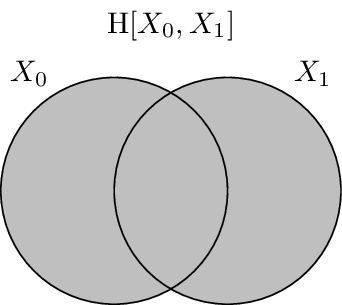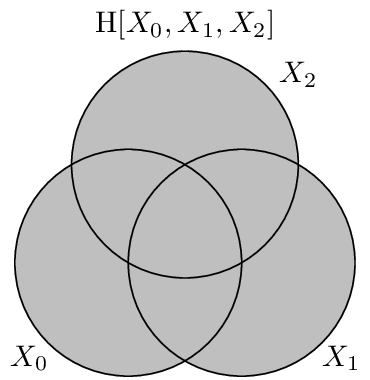# Entropy¶

The entropy measures the total amount of information contained in a set of random variables, $$X_{0:n}$$, potentially excluding the information contain in others, $$Y_{0:m}$$.

$\begin{split}\H{X_{0:n} | Y_{0:m}} = -\sum_{\substack{x_{0:n} \in \mathcal{X}_{0:n} \\ y_{0:m} \in \mathcal{Y}_{0:m}}} p(x_{0:n}, y_{0:m}) \log_2 p(x_{0:n}|y_{0:m})\end{split}$

Let’s consider two coins that are interdependent: the first coin fips fairly, and if the first comes up heads, the other is fair, but if the first comes up tails the other is certainly tails:

In : In : d = dit.Distribution(['HH', 'HT', 'TT'], [1/4, 1/4, 1/2])


We would expect that entropy of the second coin conditioned on the first coin would be $$0.5$$ bits, and sure enough that is what we find:

In : In : from dit.multivariate import entropy


And since the first coin is fair, we would expect it to have an entropy of $$1$$ bit:

In : In : entropy(d, )
Out: 1.0

In : Out: 1.0


Taken together, we would then expect the joint entropy to be $$1.5$$ bits:

In : In : entropy(d)
Out: 1.5

In : Out: 1.5


## Visualization¶

Below we have a pictoral representation of the joint entropy for both 2 and 3 variable joint distributions.## API¶

entropy(dist, rvs=None, crvs=None, rv_mode=None)[source]

Calculates the conditional joint entropy.

Parameters
• dist (Distribution) – The distribution from which the entropy is calculated.

• rvs (list, None) – The indexes of the random variable used to calculate the entropy. If None, then the entropy is calculated over all random variables.

• crvs (list, None) – The indexes of the random variables to condition on. If None, then no variables are conditioned on.

• rv_mode (str, None) – Specifies how to interpret rvs and crvs. Valid options are: {‘indices’, ‘names’}. If equal to ‘indices’, then the elements of crvs and rvs are interpreted as random variable indices. If equal to ‘names’, the the elements are interpreted as random variable names. If None, then the value of dist._rv_mode is consulted, which defaults to ‘indices’.

Returns

H – The entropy.

Return type

float

Raises

ditException – Raised if rvs or crvs contain non-existant random variables.

Examples

Let’s construct a 3-variable distribution for the XOR logic gate and name the random variables X, Y, and Z.

>>> d = dit.example_dists.Xor()
>>> d.set_rv_names(['X', 'Y', 'Z'])


The joint entropy of H[X,Y,Z] is:

>>> dit.multivariate.entropy(d, 'XYZ')
2.0


We can do this using random variables indexes too.

>>> dit.multivariate.entropy(d, [0,1,2], rv_mode='indexes')
2.0


The joint entropy H[X,Z] is given by:

>>> dit.multivariate.entropy(d, 'XZ')
1.0


Conditional entropy can be calculated by passing in the conditional random variables. The conditional entropy H[Y|X] is:

>>> dit.multivariate.entropy(d, 'Y', 'X')
1.0